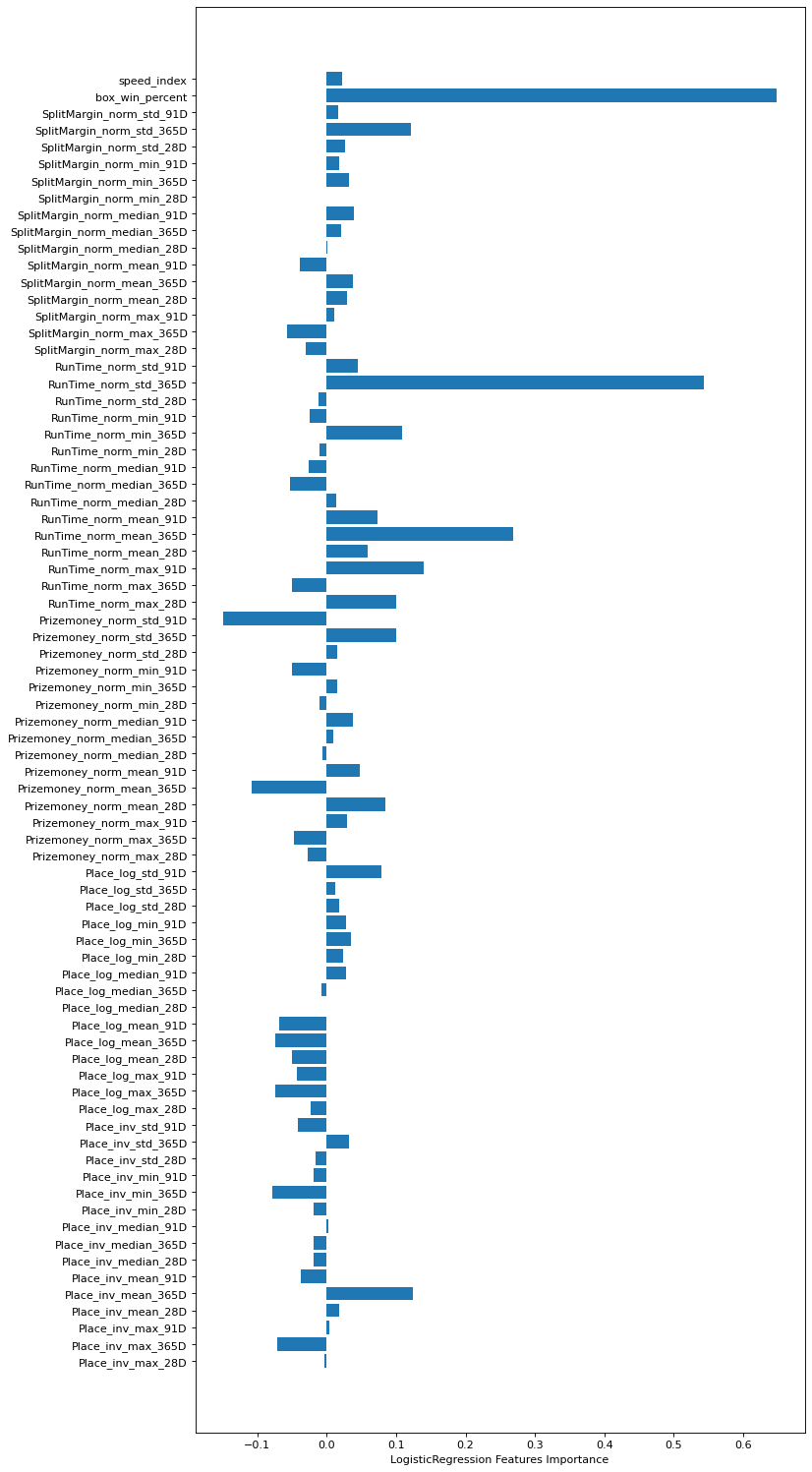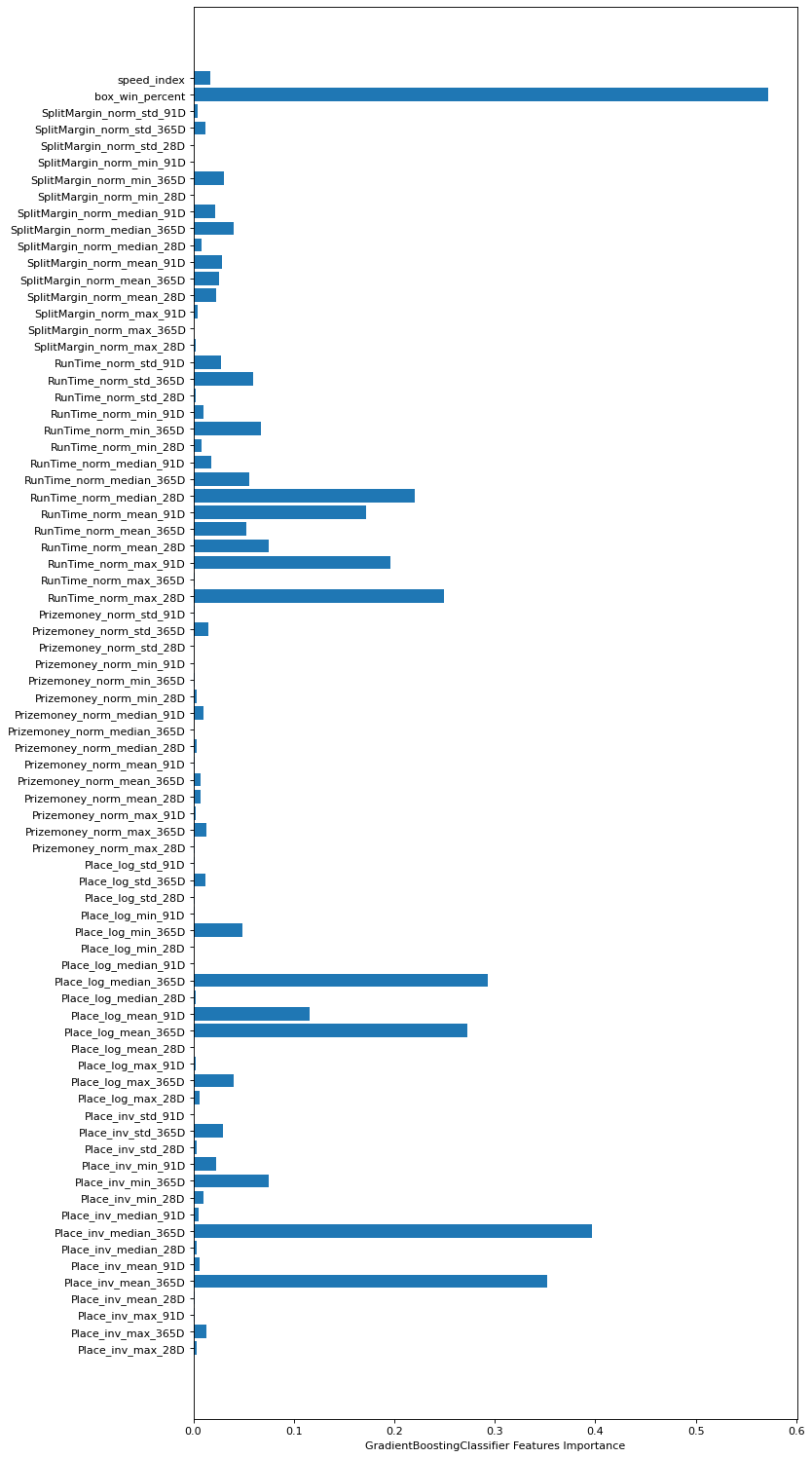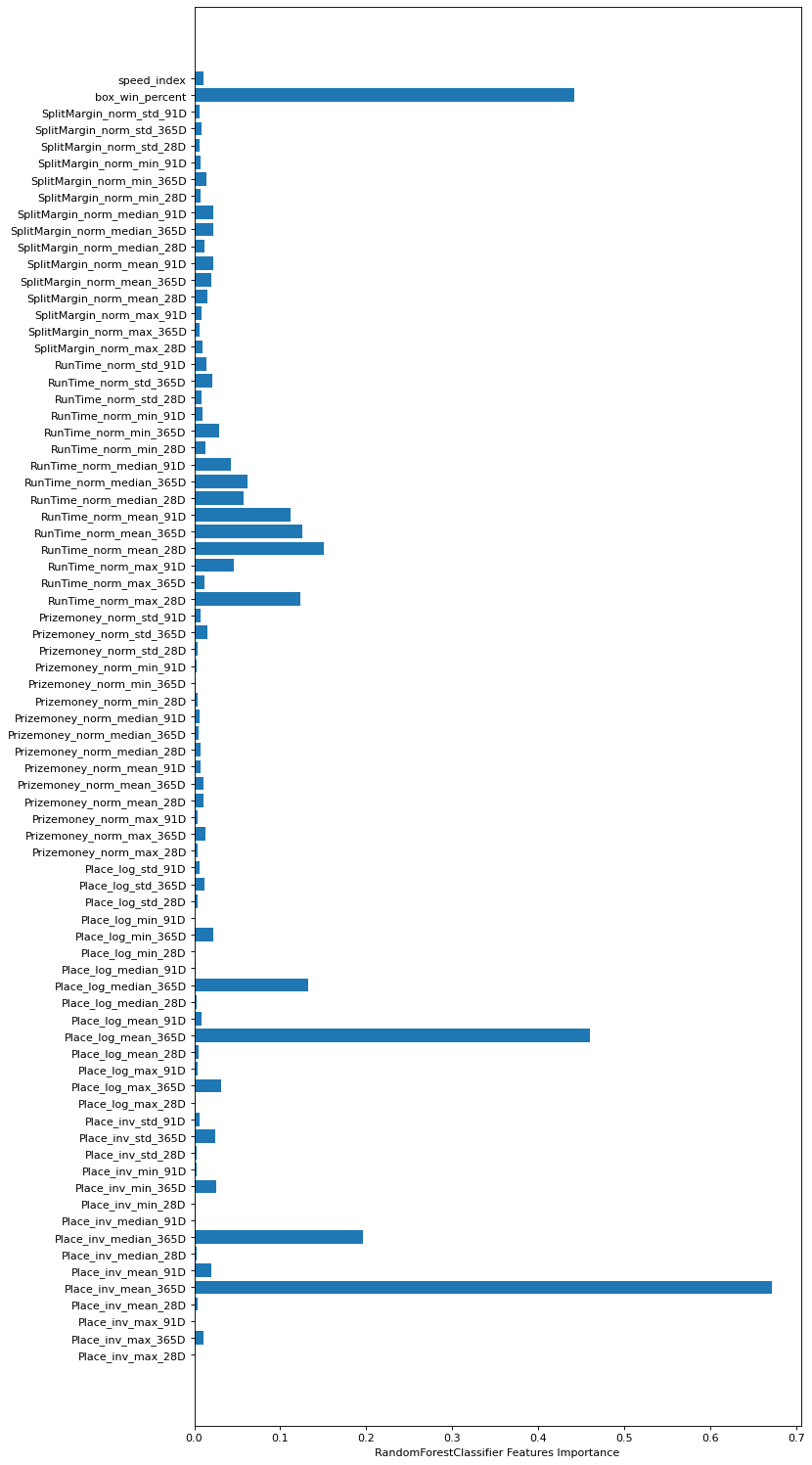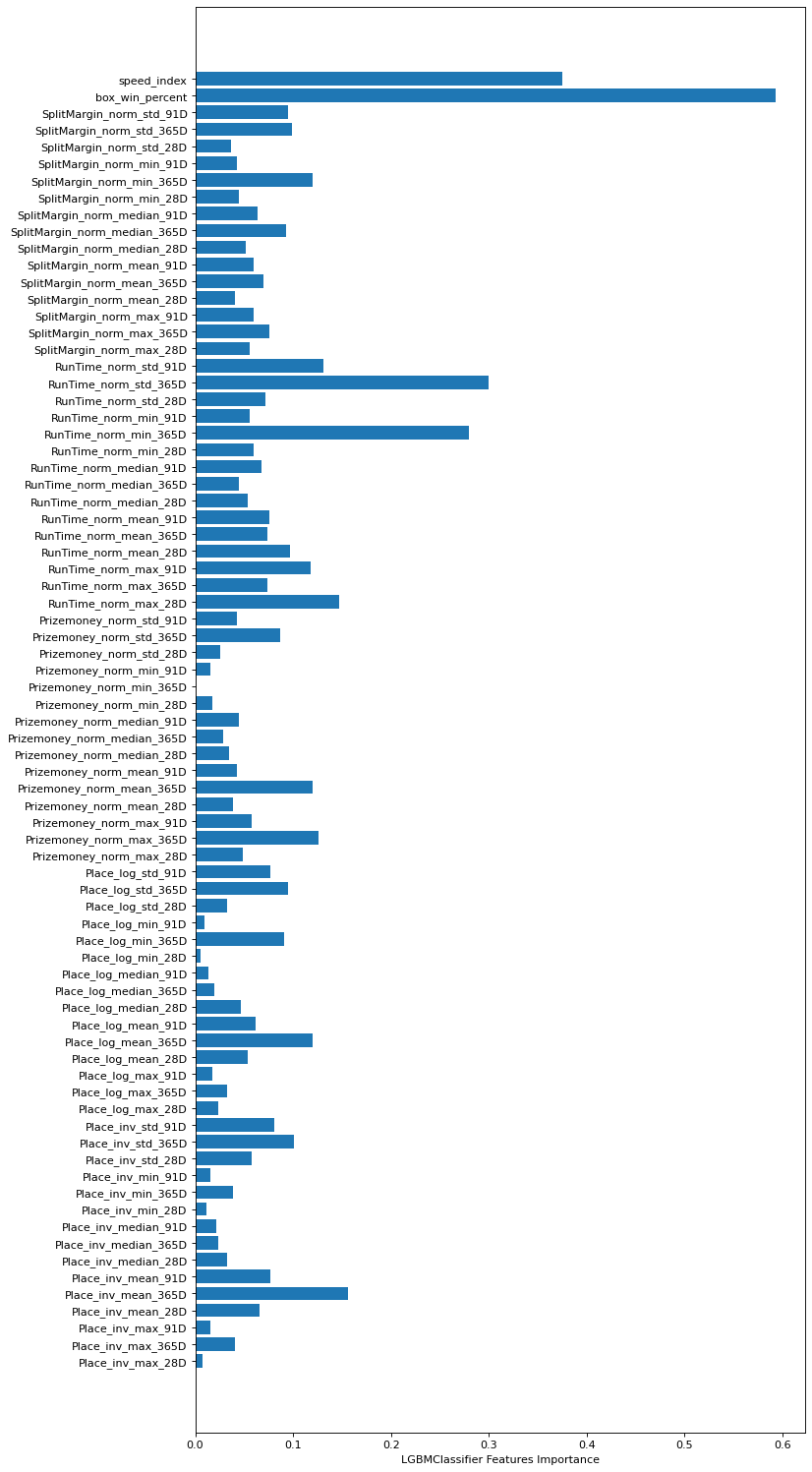# Greyhound modelling in Python

| Building a Greyhound Racing model with Scikit-learn Logistic Regression and Ensemble Learning

### Workshop

This tutorial was written by Bruno Chauvet and was originally published on Github. It is shared here with his permission.

This tutorial follows on logically from the Greyhound form Fasttrack tutorial we shared previously.

As always please reach out with feedback, suggestions or queries, or feel free to submit a pull request if you catch some bugs or have other improvements!

## Overview

This tutorial will walk you through the different steps required to generate Greyhound racing winning probabilities

2. Cleanse and normalise the data
3. Generate features using raw data
4. Build and train classification models
5. Evaluate models' performances
6. Evaluate feature importance

## Requirements

``````# Import libraries
import os
import sys

# Allow imports from src folder
module_path = os.path.abspath(os.path.join('../src'))
if module_path not in sys.path:
sys.path.append(module_path)

from datetime import datetime, timedelta
from dateutil.relativedelta import relativedelta
from dateutil import tz
from pandas.tseries.offsets import MonthEnd
from sklearn.preprocessing import MinMaxScaler
import itertools

import math
import numpy as np
import pandas as pd
import fasttrack as ft

``````
````True`
```

### Note - FastTrack API key

If you follow README instructions to run this notebook locally, you should have configured a `.env` file with your FastTrack API key. Otherwise you can set your API key below.

``````# Validate FastTrack API connection
api_key = os.getenv('FAST_TRACK_API_KEY', '<REPLACE WITH YOUR KEY>')
client = ft.Fasttrack(api_key)
track_codes = client.listTracks()
``````
``````Valid Security Key
```
```

The cell below downloads FastTrack AU race data for the past few months. Data is cached locally in the `data` folder so it can easily be reused for further processing. Depending on the amount of data to retrieve, this can take a few hours.

``````# Import race data excluding NZ races
au_tracks_filter = list(track_codes[track_codes['state'] != 'NZ']['track_code'])

# Time window to import data
# First day of the month 46 months back from now
date_from = (datetime.today() - relativedelta(months=46)).replace(day=1).strftime('%Y-%m-%d')
# First day of previous month
date_to = (datetime.today() - relativedelta(months=1)).replace(day=1).strftime('%Y-%m-%d')

# Dataframes to populate data with
race_details = pd.DataFrame()
dog_results = pd.DataFrame()

# For each month, either fetch data from API or use local CSV file if we already have downloaded it
for start in pd.date_range(date_from, date_to, freq='MS'):
start_date = start.strftime("%Y-%m-%d")
end_date = (start + MonthEnd(1)).strftime("%Y-%m-%d")
try:
filename_races = f'FT_AU_RACES_{start_date}.csv'
filename_dogs = f'FT_AU_DOGS_{start_date}.csv'

filepath_races = f'../data/{filename_races}'
filepath_dogs = f'../data/{filename_dogs}'

if os.path.isfile(filepath_races):
else:
# Fetch data from API
month_race_details, month_dog_results = client.getRaceResults(start_date, end_date, au_tracks_filter)
month_race_details.to_csv(filepath_races, index=False)
month_dog_results.to_csv(filepath_dogs, index=False)

# Combine monthly data
race_details = race_details.append(month_race_details, ignore_index=True)
dog_results = dog_results.append(month_dog_results, ignore_index=True)
except:
print(f'Could not load data from {start_date} to {end_date}')
``````
``````Loading data from 2018-11-01 to 2018-11-30
```
```

To better understand the data we retrieved, let's print the first few rows

``````# Race data
``````
@id RaceNum RaceName RaceTime Distance RaceGrade Track date
0 278896185 1 TRIPLE M BENDIGO 93.5 02:54PM 425m Grade 6 Bendigo 01 Dec 17
1 278896189 2 GOLDEN CITY CONCRETE PUMPING 03:17PM 500m Mixed 6/7 Bendigo 01 Dec 17
2 275589809 3 RAILWAY STATION HOTEL FINAL 03:38PM 500m Mixed 6/7 Final Bendigo 01 Dec 17
3 278896183 4 MCIVOR RD VETERINARY CLINIC 03:58PM 425m Grade 5 Bendigo 01 Dec 17
4 278896179 5 GRV VIC BRED SERIES HT1 04:24PM 425m Grade 5 Heat Bendigo 01 Dec 17
``````# Individual dogs results
``````
@id Place DogName Box Rug Weight StartPrice Handicap Margin1 Margin2 PIR Checks Comments SplitMargin RunTime Prizemoney RaceId TrainerId TrainerName
0 124886334 1 VANDA MICK 2.0 2 32.0 \$2.80F NaN 0.49 NaN S/231 0 NaN 6.79 24.66 NaN 278896185 66993 M Ellis
1 2027130024 2 DYNA ZAD 7.0 7 24.2 \$6.60 NaN 0.49 0.49 M/843 4 NaN 6.95 24.69 NaN 278896185 115912 M Delbridge
2 1448760015 3 KLONDIKE GOLD 4.0 4 33.3 \$16.60 NaN 1.83 1.34 M/422 0 NaN 6.81 24.79 NaN 278896185 94459 R Hayes
3 1449650024 4 FROSTY TIARA 3.0 3 26.8 \$22.00 NaN 2.94 1.11 S/114 0 NaN 6.75 24.86 NaN 278896185 87428 R Morgan
4 118782592 5 GNOCCHI 1.0 1 29.6 \$8.60 NaN 6.50 3.56 S/355 0 NaN 6.80 25.11 NaN 278896185 138164 J La Rosa

From the FastTrack documentation, this is what each variable represents:

Variable Description
Box Integer value between 1 and 8
Rug This is an integer value between 1 and 10
Weight This is a decimal value to 1 decimal place
StartPrice This is an integer value > 0 that is prefixed by the character "\$"
Handicap Empty = Not a Handicapped Race "Y" = Handicapped Race
Margin1 This is a decimal value to two decimal places representing a dogs margin from the winning dog, in the case of the winning dog, it is the margin to the second dog.
Margin2 This is a decimal value to two decimal places representing a dogs margin from the dog in front if it, in the case of the winning dog this value is empty.
PIR This is a dogs place at each of the split points in a race. The string representation is \/\\\
The Speed values will be one of the following:
S = “Slow Start”
M = “Medium Start”
F = “Fast Start”
E.g. S/444 = Slow start and was placed 4th at each of the races
three split points
Checks Whether the dog was checked in running by other dogs, and the number of lengths lost as a result of the checking, ie. C1
SplitMargin This is a decimal value to two decimal places representing a dogs time at the first split marker.
RunTime This is a decimal value to two decimal places representing a dogs running time for a race
PriceMoney This is an integer value > 0 with a “\$” character prefix. Maximum of 8 values

## 2. Cleanse and normalise the data

Here we do some basic data manipulation and cleansing to get variables into format that we can work with

``````# Clean up the race dataset
race_details = race_details.rename(columns = {'@id': 'FastTrack_RaceId'})
race_details['Distance'] = race_details['Distance'].apply(lambda x: int(x.replace("m", "")))
race_details['date_dt'] = pd.to_datetime(race_details['date'], format = '%d %b %y')
``````
``````# Clean up the dogs results dataset
dog_results = dog_results.rename(columns = {'@id': 'FastTrack_DogId', 'RaceId': 'FastTrack_RaceId'})

# Combine dogs results with race attributes
dog_results = dog_results.merge(
how = 'left',
on = 'FastTrack_RaceId'
)

# Convert StartPrice to probability
dog_results['StartPrice'] = dog_results['StartPrice'].apply(lambda x: None if x is None else float(x.replace('\$', '').replace('F', '')) if isinstance(x, str) else x)
dog_results['StartPrice_probability'] = (1 / dog_results['StartPrice']).fillna(0)
dog_results['StartPrice_probability'] = dog_results.groupby('FastTrack_RaceId')['StartPrice_probability'].apply(lambda x: x / x.sum())

# Discard entries without results (scratched or did not finish)
dog_results = dog_results[~dog_results['Box'].isnull()]
dog_results['Box'] = dog_results['Box'].astype(int)

# Clean up other attributes
dog_results['RunTime'] = dog_results['RunTime'].astype(float)
dog_results['SplitMargin'] = dog_results['SplitMargin'].astype(float)
dog_results['Prizemoney'] = dog_results['Prizemoney'].astype(float).fillna(0)
dog_results['Place'] = pd.to_numeric(dog_results['Place'].apply(lambda x: x.replace("=", "") if isinstance(x, str) else 0), errors='coerce').fillna(0)
dog_results['win'] = dog_results['Place'].apply(lambda x: 1 if x == 1 else 0)
``````

The cell below shows some normalisation techniques. Why normalise data? Microsoft Azure has an excellent article on why this technique is often applied.

• Apply `Log base 10` transformation to `Prizemoney` and `Place`
• Apply `inverse` transformation to `Place`
• Combine `RunTime` and `Distance` to generate `Speed` value
``````# Normalise some of the raw values
dog_results['Prizemoney_norm'] = np.log10(dog_results['Prizemoney'] + 1) / 12
dog_results['Place_inv'] = (1 / dog_results['Place']).fillna(0)
dog_results['Place_log'] = np.log10(dog_results['Place'] + 1).fillna(0)
dog_results['RunSpeed'] = (dog_results['RunTime'] / dog_results['Distance']).fillna(0)
``````

## 3. Generate features using raw data

### Calculate median winner time by track/distance

To compare individual runner times, we extract the median winner time for each Track/Distance and use it as a reference time.

``````# Calculate median winner time per track/distance
win_results = dog_results[dog_results['win'] == 1]
median_win_time = pd.DataFrame(data=win_results[win_results['RunTime'] > 0].groupby(['Track', 'Distance'])['RunTime'].median()).rename(columns={"RunTime": "RunTime_median"}).reset_index()
median_win_split_time = pd.DataFrame(data=win_results[win_results['SplitMargin'] > 0].groupby(['Track', 'Distance'])['SplitMargin'].median()).rename(columns={"SplitMargin": "SplitMargin_median"}).reset_index()
``````
Track Distance RunTime_median
0 Albion Park 331 19.180
1 Albion Park 395 22.860
2 Albion Park 520 30.220
3 Albion Park 600 35.100
4 Albion Park 710 42.005

### Calculate Track speed index

Some tracks are run faster than other, we calculate here a `speed_index` using the track reference time over the travelled distance. The lower the `speed_index`, the faster the track is. We use `MinMaxScaler` to scale `speed_index` values between zero and one.

``````# Calculate track speed index
median_win_time['speed_index'] = (median_win_time['RunTime_median'] / median_win_time['Distance'])
median_win_time['speed_index'] = MinMaxScaler().fit_transform(median_win_time[['speed_index']])
``````
Track Distance RunTime_median speed_index
0 Albion Park 331 19.180 0.471787
1 Albion Park 395 22.860 0.460736
2 Albion Park 520 30.220 0.497773
3 Albion Park 600 35.100 0.556644
4 Albion Park 710 42.005 0.657970

### Compare individual times with track reference time

For each dog result, we compare the runner time with the reference time using the formula `(track reference time) / (runner time)` and normalise the result. The higher the value, the quicker the dog was.

``````# Compare dogs finish time with median winner time
dog_results = dog_results.merge(median_win_time, on=['Track', 'Distance'], how='left')
dog_results = dog_results.merge(median_win_split_time, on=['Track', 'Distance'], how='left')

# Normalise time comparison
dog_results['RunTime_norm'] = (dog_results['RunTime_median'] / dog_results['RunTime']).clip(0.9, 1.1)
dog_results['RunTime_norm'] = MinMaxScaler().fit_transform(dog_results[['RunTime_norm']])
dog_results['SplitMargin_norm'] = (dog_results['SplitMargin_median'] / dog_results['SplitMargin']).clip(0.9, 1.1)
dog_results['SplitMargin_norm'] = MinMaxScaler().fit_transform(dog_results[['SplitMargin_norm']])
``````
FastTrack_DogId Place DogName Box Rug Weight StartPrice Handicap Margin1 Margin2 ... win Prizemoney_norm Place_inv Place_log RunSpeed RunTime_median speed_index SplitMargin_median RunTime_norm SplitMargin_norm
0 124886334 1.0 VANDA MICK 2 2 32.0 2.8 NaN 0.49 NaN ... 1 0.0 1.000000 0.301030 0.058024 24.21 0.321642 6.63 0.408759 0.382180
1 2027130024 2.0 DYNA ZAD 7 7 24.2 6.6 NaN 0.49 0.49 ... 0 0.0 0.500000 0.477121 0.058094 24.21 0.321642 6.63 0.402795 0.269784
2 1448760015 3.0 KLONDIKE GOLD 4 4 33.3 16.6 NaN 1.83 1.34 ... 0 0.0 0.333333 0.602060 0.058329 24.21 0.321642 6.63 0.383017 0.367841
3 1449650024 4.0 FROSTY TIARA 3 3 26.8 22.0 NaN 2.94 1.11 ... 0 0.0 0.250000 0.698970 0.058494 24.21 0.321642 6.63 0.369268 0.411111
4 118782592 5.0 GNOCCHI 1 1 29.6 8.6 NaN 6.50 3.56 ... 0 0.0 0.200000 0.778151 0.059082 24.21 0.321642 6.63 0.320789 0.375000

5 rows × 34 columns

### Barrier winning probabilities

The barrier dogs start from play a big part in the race so we calculate the winning percentage for each `barrier/track/distance`

``````# Calculate box winning percentage for each track/distance
box_win_percent = pd.DataFrame(data=dog_results.groupby(['Track', 'Distance', 'Box'])['win'].mean()).rename(columns={"win": "box_win_percent"}).reset_index()
# Add to dog results dataframe
dog_results = dog_results.merge(box_win_percent, on=['Track', 'Distance', 'Box'], how='left')
# Display example of barrier winning probabilities
``````
Track Distance Box box_win_percent
0 Albion Park 331 1 0.195652
1 Albion Park 331 2 0.153472
2 Albion Park 331 3 0.125446
3 Albion Park 331 4 0.124615
4 Albion Park 331 5 0.116135
5 Albion Park 331 6 0.105144
6 Albion Park 331 7 0.104770
7 Albion Park 331 8 0.115095

### Generate time-based features

Now that we have a set of basic features for individual dog results, we need to aggregate them into a single feature vector.

To do so, we calculate the `min`, `max`, `mean`, `median`, `std` of features previously caculated over different time windows `28`, `91` and `365` days:

• `RunTime_norm`
• `SplitMargin_norm`
• `Place_inv`
• `Place_log`
• `Prizemoney_norm`

This will allow us to create a short, medium and long term measures of our features.

Depending on the dataset size, this can take several minutes.

``````# Generate rolling window features
dataset = dog_results.copy()
dataset = dataset.set_index(['FastTrack_DogId', 'date_dt']).sort_index()

# Use rolling window of 28, 91 and 365 days
rolling_windows = ['28D', '91D', '365D']
# Features to use for rolling windows calculation
features = ['RunTime_norm', 'SplitMargin_norm', 'Place_inv', 'Place_log', 'Prizemoney_norm']
# Aggregation functions to apply
aggregates = ['min', 'max', 'mean', 'median', 'std']
# Keep track of generated feature names
feature_cols = ['speed_index', 'box_win_percent']

for rolling_window in rolling_windows:
print(f'Processing rolling window {rolling_window}')

rolling_result = (
dataset
.reset_index(level=0)
.groupby('FastTrack_DogId')[features]
.rolling(rolling_window)
.agg(aggregates)
.groupby(level=0)
.shift(1)
)

# Generate list of rolling window feature names (eg: RunTime_norm_min_365D)
agg_features_cols = [f'{f}_{a}_{rolling_window}' for f, a in itertools.product(features, aggregates)]
dataset[agg_features_cols] = rolling_result
# Keep track of generated feature names
feature_cols.extend(agg_features_cols)
``````
``````Processing rolling window 28D
Processing rolling window 91D
Processing rolling window 365D
```
```
``````# Replace missing values with 0
dataset.fillna(0, inplace=True)
``````
Place DogName Box Rug Weight StartPrice Handicap Margin1 Margin2 PIR ... Place_log_min_365D Place_log_max_365D Place_log_mean_365D Place_log_median_365D Place_log_std_365D Prizemoney_norm_min_365D Prizemoney_norm_max_365D Prizemoney_norm_mean_365D Prizemoney_norm_median_365D Prizemoney_norm_std_365D
FastTrack_DogId date_dt
-2143487296 2017-12-14 6.0 JEWELLED COIN 7 7 26.6 13.1 0.0 8.25 0.14 55 ... 0.000000 0.000000 0.000000 0.000000 0.000000 0.0 0.000000 0.000000 0.000000 0.000000
2017-12-21 4.0 JEWELLED COIN 5 5 26.8 9.7 0.0 13.50 3.00 555 ... 0.845098 0.845098 0.845098 0.845098 0.000000 0.0 0.000000 0.000000 0.000000 0.000000
2017-12-26 3.0 JEWELLED COIN 3 3 27.1 21.5 0.0 6.75 2.29 642 ... 0.698970 0.845098 0.772034 0.772034 0.103328 0.0 0.142298 0.071149 0.071149 0.100620
2017-12-30 7.0 JEWELLED COIN 7 9 26.4 48.1 0.0 21.75 2.29 7777 ... 0.602060 0.845098 0.715376 0.698970 0.122347 0.0 0.204697 0.115665 0.142298 0.104915
2018-01-02 8.0 JEWELLED COIN 5 5 26.8 32.7 0.0 15.75 0.00 888 ... 0.602060 0.903090 0.762305 0.772034 0.137070 0.0 0.204697 0.086749 0.071149 0.103357
2018-01-08 4.0 JEWELLED COIN 1 1 27.2 2.5 0.0 6.50 1.29 5443 ... 0.602060 0.954243 0.800692 0.845098 0.146490 0.0 0.204697 0.069399 0.000000 0.097556
2018-01-10 2.0 JEWELLED COIN 5 5 27.3 8.5 0.0 2.00 2.14 442 ... 0.602060 0.954243 0.783738 0.772034 0.137448 0.0 0.204697 0.080926 0.069282 0.091711
2018-01-17 2.0 JEWELLED COIN 3 3 27.4 7.3 0.0 5.25 5.14 433 ... 0.477121 0.954243 0.739936 0.698970 0.170804 0.0 0.204697 0.098329 0.138563 0.095547

8 rows × 108 columns

As we use up to a year of data to generate our feature set, we exclude the first year of the dataset from our training dataset

``````# Only keep data after 2018-12-01
model_df = dataset.reset_index()
feature_cols = np.unique(feature_cols).tolist()
model_df = model_df[model_df['date_dt'] >= '2018-12-01']
model_df = model_df[['date_dt', 'FastTrack_RaceId', 'DogName', 'win', 'StartPrice_probability'] + feature_cols]

# Only train model off of races where each dog has a value for each feature
races_exclude = model_df[model_df.isnull().any(axis = 1)]['FastTrack_RaceId'].drop_duplicates()
model_df = model_df[~model_df['FastTrack_RaceId'].isin(races_exclude)]
``````

## 4. Build and train regression models

### Logistic regression

The dataset is split between training and validation:

• train dataset: from 2018-12-01 to 2020-12-31
• validation dataset: from 2021-01-01

The next cell trains a LogisticRegression model and uses the `win` flag (`0=lose, 1=win`) as a target.

``````from matplotlib import pyplot
from matplotlib.pyplot import figure

from sklearn.linear_model import LogisticRegression

# Split the data into train and test data
train_data = model_df[model_df['date_dt'] < '2021-01-01'].reset_index(drop = True).sample(frac=1)
test_data = model_df[model_df['date_dt'] >= '2021-01-01'].reset_index(drop = True)

# Use our previously built features set columns as Training vector
# Use win flag as Target vector
train_x, train_y = train_data[feature_cols], train_data['win']
test_x, test_y = test_data[feature_cols], test_data['win']

# Build a LogisticRegression model
model = LogisticRegression(verbose=0, solver='saga', n_jobs=-1)

# Train the model
print(f'Training on {len(train_x):,} samples with {len(feature_cols)} features')
model.fit(train_x, train_y)
``````
``````Training on 630,306 samples with 77 features
```
```
````LogisticRegression(n_jobs=-1, solver='saga')`
```

## 5. Evaluate model predictions

Now that we have trained our model, we can generate predictions on the test dataset

``````# Generate runner win predictions
dog_win_probabilities = model.predict_proba(test_x)[:,1]
test_data['prob_LogisticRegression'] = dog_win_probabilities
# Normalise probabilities
test_data['prob_LogisticRegression'] = test_data.groupby('FastTrack_RaceId')['prob_LogisticRegression'].apply(lambda x: x / sum(x))
``````

### Model strike rate

Knowing how often a model correctly predicts the winner is one of the most important metrics

``````# Create a boolean column for whether a dog has the higehst model prediction in a race
test_dataset_size = test_data['FastTrack_RaceId'].nunique()
odds_win_prediction = test_data.groupby('FastTrack_RaceId')['prob_LogisticRegression'].apply(lambda x: x == max(x))
odds_win_prediction_percent = len(test_data[(odds_win_prediction == True) & (test_data['win'] == 1)]) / test_dataset_size
print(f"LogisticRegression strike rate: {odds_win_prediction_percent:.2%}")
``````
``````LogisticRegression strike rate: 32.57%
```
```

### Brier score

The Brier score measures the mean squared difference between the predicted probability and the actual outcome. The smaller the Brier score loss, the better.

``````from sklearn.metrics import brier_score_loss

brier_score = brier_score_loss(test_data['win'], test_data['prob_LogisticRegression'])
print(f'LogisticRegression Brier score: {brier_score:.8}')
``````
``````LogisticRegression Brier score: 0.11074995
```
```

### Predictions' distribution

To get a better feel of what our models are predicting, we can plot the generated probabilities' distribution and compare them with Start Prices probabilities' distribution.

``````import matplotlib.pyplot as plt
import seaborn as sns

bins = 100

sns.displot(data=[test_data['prob_LogisticRegression'], test_data['StartPrice_probability']], kind="hist",
bins=bins, height=7, aspect=2)
plt.title('StartPrice vs LogisticRegression probabilities distribution')
plt.xlabel('Probability')
plt.show()
``````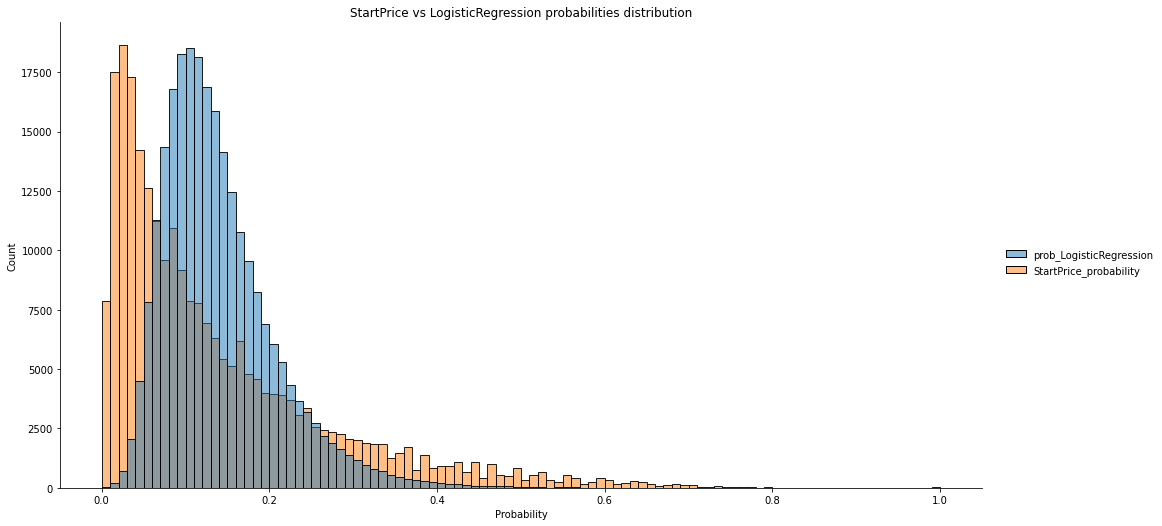Probabilities generated by the logistic regression model follow a slightly different distribution. `Scikit-learn` framework offers various hyper parameters to fine tune a model and achieve better performances.

### Predictions calibration

We want to ensure that probabilities generated by our model match real world probabilities. Calibration curves help us understand if a model needs to be calibrated.

``````from sklearn.calibration import calibration_curve

bins = 100
fig = plt.figure(figsize=(12, 9))

# Generate calibration curves based on our probabilities
cal_y, cal_x = calibration_curve(test_data['win'], test_data['prob_LogisticRegression'], n_bins=bins)

# Plot against reference line
plt.plot(cal_x, cal_y, marker='o', linewidth=1)
plt.plot([0, 1], [0, 1], '--', color='gray')
plt.title("LogisticRegression calibration curve");
``````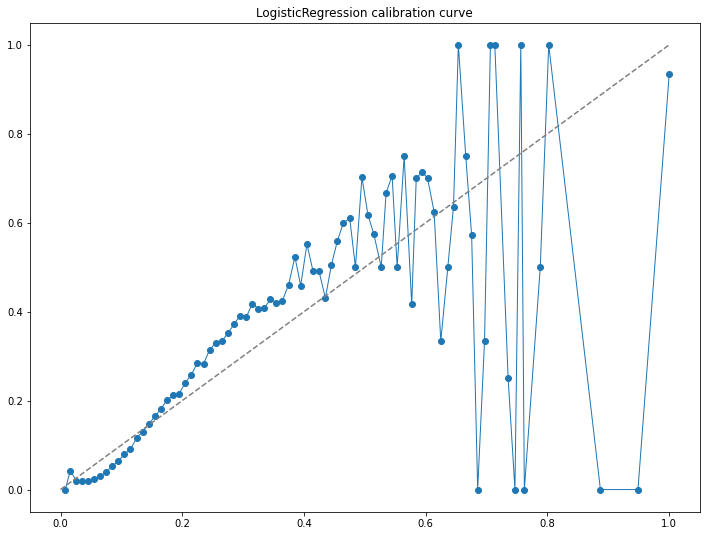A model is perfectly calibrated if the grouped values (bins) follow the dotted line. Our model generates probabilities that need to be calibrated. To get our model to generate more accurate probabilities, we would need to generate better features, test various modelling approaches and calibrate generated probabilities.

### Compare other types of classification models

The next cell trains different classification models using `Scikit-learn` unified API:

Depending on dataset size and compute capacity, this can take several minutes

``````from matplotlib import pyplot
from matplotlib.pyplot import figure

from sklearn.ensemble import RandomForestClassifier

from lightgbm import LGBMClassifier
from xgboost import XGBClassifier
from catboost import CatBoostClassifier

# Common models parameters
verbose       = 0
learning_rate = 0.1
n_estimators  = 100

# Train different types of models
models = {
'LogisticRegression':         LogisticRegression(verbose=0, solver='saga', n_jobs=-1),
'RandomForestClassifier':     RandomForestClassifier(verbose=verbose, n_estimators=n_estimators, max_depth=8, max_features=0.5, n_jobs=-1),
'LGBMClassifier':             LGBMClassifier(verbose=verbose, learning_rate=learning_rate, n_estimators=n_estimators, force_col_wise=True),
'XGBClassifier':              XGBClassifier(verbosity=verbose, learning_rate=learning_rate, n_estimators=n_estimators, objective='binary:logistic', use_label_encoder=False),
'CatBoostClassifier':         CatBoostClassifier(verbose=verbose, learning_rate=learning_rate, n_estimators=n_estimators)
}

print(f'Training on {len(train_x):,} samples with {len(feature_cols)} features')
for key, model in models.items():
print(f'Fitting model {key}')
model.fit(train_x, train_y)
``````
``````Training on 630,306 samples with 77 features
Fitting model LogisticRegression
Fitting model RandomForestClassifier
Fitting model LGBMClassifier
Fitting model XGBClassifier
Fitting model CatBoostClassifier
```
```
``````# Calculate probabilities for each model on the test dataset
probs_columns = ['StartPrice_probability']
for key, model in models.items():
probs_column_key = f'prob_{key}'
# Calculate runner win probability
dog_win_probs = model.predict_proba(test_x)[:,1]
test_data[probs_column_key] = dog_win_probs
# Normalise probabilities
test_data[probs_column_key] = test_data.groupby('FastTrack_RaceId')[f'prob_{key}'].apply(lambda x: x / sum(x))
probs_columns.append(probs_column_key)
``````

### Calculate models strike rate and Brier score

Here we compare the strike rate of the different models' predictions with the start price strike rate.

``````# Create a boolean column for whether a dog has the higehst model prediction in a race.
# Do the same for the starting price as a comparison
test_dataset_size = test_data['FastTrack_RaceId'].nunique()
odds_win_prediction = test_data.groupby('FastTrack_RaceId')['StartPrice_probability'].apply(lambda x: x == max(x))
odds_win_prediction_percent = len(test_data[(odds_win_prediction == True) & (test_data['win'] == 1)]) / test_dataset_size
brier_score = brier_score_loss(test_data['win'], test_data['StartPrice_probability'])
print(f'Starting Price                strike rate: {odds_win_prediction_percent:.2%} Brier score: {brier_score:.8}')

for key, model in models.items():
predicted_winners = test_data.groupby('FastTrack_RaceId')[f'prob_{key}'].apply(lambda x: x == max(x))
strike_rate = len(test_data[(predicted_winners == True) & (test_data['win'] == 1)]) / test_data['FastTrack_RaceId'].nunique()
brier_score = brier_score_loss(test_data['win'], test_data[f'prob_{key}'])
print(f'{key.ljust(30)}strike rate: {strike_rate:.2%} Brier score: {brier_score:.8}')
``````
``````Starting Price                strike rate: 42.24% Brier score: 0.1008106
LogisticRegression            strike rate: 32.57% Brier score: 0.11074995
GradientBoostingClassifier    strike rate: 33.31% Brier score: 0.1105322
RandomForestClassifier        strike rate: 33.24% Brier score: 0.11110442
LGBMClassifier                strike rate: 33.40% Brier score: 0.11024272
XGBClassifier                 strike rate: 33.45% Brier score: 0.11019414
CatBoostClassifier            strike rate: 33.33% Brier score: 0.11038785
```
```

### Visualise models predictions

Here we generate some probabilities using our trained models' and compare them with the start price.

In blue the lowest prediction and in red the highest prediction generated by the different models.

``````# Display and format sample results
def highlight_max(s, props=''):
return np.where(s == np.nanmax(s.values), props, '')
def highlight_min(s, props=''):
return np.where(s == np.nanmin(s.values), props, '')

test_data[probs_columns].sample(20).style \
.bar(color='#FFA07A', vmin=0.01, vmax=0.25, axis=1) \
.apply(highlight_max, props='color:red;', axis=1) \
.apply(highlight_min, props='color:blue;', axis=1)
``````
StartPrice_probability prob_LogisticRegression prob_GradientBoostingClassifier prob_RandomForestClassifier prob_LGBMClassifier prob_XGBClassifier prob_CatBoostClassifier
103796 0.168011 0.229477 0.213527 0.198978 0.212484 0.201237 0.216716
148438 0.099749 0.094426 0.128795 0.157217 0.111212 0.064149 0.125434
47999 0.013440 0.063647 0.070135 0.096175 0.077220 0.079547 0.079643
226804 0.331895 0.074406 0.093237 0.087665 0.070858 0.074815 0.092281
14964 0.025668 0.031759 0.028652 0.037364 0.028186 0.025395 0.025159
139208 0.537243 0.269383 0.304885 0.300394 0.286015 0.301443 0.296410
43027 0.257494 0.137246 0.114401 0.113054 0.093332 0.108154 0.126703
151933 0.254678 0.280246 0.204094 0.188197 0.211623 0.196675 0.219325
75586 0.126036 0.106833 0.100814 0.115142 0.111017 0.118383 0.107643
114240 0.530708 0.162428 0.159064 0.129457 0.188566 0.172335 0.153399
73858 0.158025 0.228463 0.233774 0.228696 0.221210 0.217358 0.230763
174698 0.040139 0.039564 0.045854 0.048556 0.040328 0.039350 0.042728
121778 0.036096 0.084464 0.092160 0.097381 0.084467 0.088923 0.089644
227215 0.046275 0.088997 0.090732 0.109514 0.109888 0.109474 0.105836
10731 0.074040 0.106214 0.076128 0.080542 0.070221 0.072588 0.080146
88484 0.064234 0.120542 0.104910 0.117783 0.134223 0.145592 0.114074
104690 0.064302 0.145388 0.126006 0.141474 0.129206 0.120031 0.128490
201852 0.602269 0.367840 0.394838 0.364498 0.401240 0.387149 0.393707
218537 0.073158 0.121725 0.115405 0.113319 0.116149 0.098413 0.112932
119928 0.310934 0.191449 0.176635 0.162701 0.187676 0.182536 0.154486

We now have built a simple feature set and trained models using various classification techniques. To improve our model's performance, one should build a more advanced feature set and fine tune the model's hyper parameters.

## 6. Display models' features' importance

``````from sklearn.preprocessing import normalize

total_feature_importances = []

# Individual models feature importance
for key, model in models.items():
figure(figsize=(10, 24), dpi=80)
if isinstance(model, LogisticRegression):
feature_importance = model.coef_
else:
feature_importance = model.feature_importances_

feature_importance = normalize(feature_importance[:,np.newaxis], axis=0).ravel()
total_feature_importances.append(feature_importance)
pyplot.barh(feature_cols, feature_importance)
pyplot.xlabel(f'{key} Features Importance')
pyplot.show()

# Overall feature importance
avg_feature_importances = np.asarray(total_feature_importances).mean(axis=0)
figure(figsize=(10, 24), dpi=80)
pyplot.barh(feature_cols, avg_feature_importances)
pyplot.xlabel('Overall Features Importance')
pyplot.show()
``````